Prealgebra

# 11.3Graphing with Intercepts

Prealgebra11.3 Graphing with Intercepts

### Learning Objectives

By the end of this section, you will be able to:
• Identify the intercepts on a graph
• Find the intercepts from an equation of a line
• Graph a line using the intercepts
• Choose the most convenient method to graph a line

### Be Prepared 11.3

Before you get started, take this readiness quiz.

1. Solve: $3x+4y=−123x+4y=−12$ for $xx$ when $y=0.y=0.$
If you missed this problem, review Example 9.62.
2. Is the point $(0,−5)(0,−5)$ on the $x-axisx-axis$ or $y-axis?y-axis?$
If you missed this problem, review Example 11.5.
3. Which ordered pairs are solutions to the equation $2x−y=6?2x−y=6?$
$(6,0)(6,0)$$(0,−6)(0,−6)$$(4,−2).(4,−2).$
If you missed this problem, review Example 11.8.

### Identify the Intercepts on a Graph

Every linear equation has a unique line that represents all the solutions of the equation. When graphing a line by plotting points, each person who graphs the line can choose any three points, so two people graphing the line might use different sets of points.

At first glance, their two lines might appear different since they would have different points labeled. But if all the work was done correctly, the lines will be exactly the same line. One way to recognize that they are indeed the same line is to focus on where the line crosses the axes. Each of these points is called an intercept of the line.

### Intercepts of a Line

Each of the points at which a line crosses the $x-axisx-axis$ and the $y-axisy-axis$ is called an intercept of the line.

Let’s look at the graph of the lines shown in Figure 11.14.

Figure 11.14

First, notice where each of these lines crosses the x- axis:

Figure: The line crosses the x-axis at: Ordered pair of this point
42 3 (3,0)
43 4 (4,0)
44 5 (5,0)
45 0 (0,0)

Do you see a pattern?

For each row, the y- coordinate of the point where the line crosses the x- axis is zero. The point where the line crosses the x- axis has the form $(a,0)(a,0)$; and is called the x-intercept of the line. The x- intercept occurs when y is zero.

Now, let's look at the points where these lines cross the y-axis.

Figure: The line crosses the y-axis at: Ordered pair for this point
42 6 (0,6)
43 -3 (0,-3)
44 -5 (0,-5)
45 0 (0,0)

### x- intercept and y- intercept of a line

The $x-interceptx-intercept$ is the point, $(a, 0),(a,0),$ where the graph crosses the $x-axis.x-axis.$ The $x-interceptx-intercept$ occurs when $yy$ is zero.

The $y-intercepty-intercept$ is the point, $(0, b),(0,b),$ where the graph crosses the $y-axis.y-axis.$

The $y-intercepty-intercept$ occurs when $xx$ is zero.

### Example 11.23

Find the $x- andy-interceptsx- andy-intercepts$ of each line:

 ⓐ$x+2y=4x+2y=4$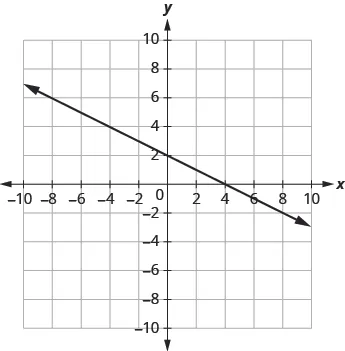ⓑ$3x−y=63x−y=6$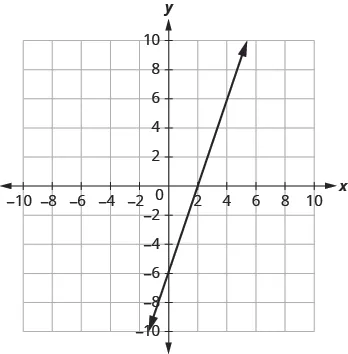ⓒ$x+y=−5x+y=−5$### Try It 11.44

Find the $x-x-$ and $y-interceptsy-intercepts$ of the graph: $x−y=2.x−y=2.$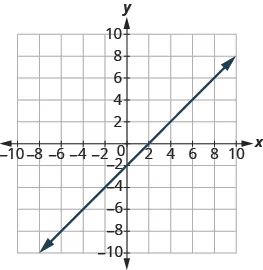### Try It 11.45

Find the $x-x-$ and $y-interceptsy-intercepts$ of the graph: $2x+3y=6.2x+3y=6.$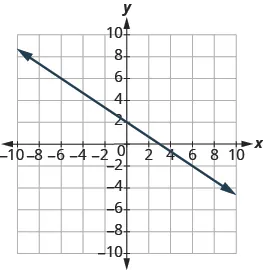### Find the Intercepts from an Equation of a Line

Recognizing that the $x-interceptx-intercept$ occurs when $yy$ is zero and that the $y-intercepty-intercept$ occurs when $xx$ is zero gives us a method to find the intercepts of a line from its equation. To find the $x-intercept,x-intercept,$ let $y=0y=0$ and solve for $x.x.$ To find the $y-intercept,y-intercept,$ let $x=0x=0$ and solve for $y.y.$

### Find the x and y from the Equation of a Line

Use the equation to find:

• the x-intercept of the line, let $y=0y=0$ and solve for x.
• the y-intercept of the line, let $x=0x=0$ and solve for y.
x y
0
0

### Example 11.24

Find the intercepts of $2x+y=62x+y=6$

### Try It 11.46

Find the intercepts: $3x+y=123x+y=12$

### Try It 11.47

Find the intercepts: $x+4y=8x+4y=8$

### Example 11.25

Find the intercepts of $4x−3y=12.4x−3y=12.$

### Try It 11.48

Find the intercepts of the line: $3x−4y=12.3x−4y=12.$

### Try It 11.49

Find the intercepts of the line: $2x−4y=8.2x−4y=8.$

### Graph a Line Using the Intercepts

To graph a linear equation by plotting points, you can use the intercepts as two of your three points. Find the two intercepts, and then a third point to ensure accuracy, and draw the line. This method is often the quickest way to graph a line.

### Example 11.26

Graph $−x+2y=6−x+2y=6$ using intercepts.

### Try It 11.50

Graph the line using the intercepts: $x−2y=4.x−2y=4.$

### Try It 11.51

Graph the line using the intercepts: $−x+3y=6.−x+3y=6.$

### How To

#### Graph a line using the intercepts.

1. Step 1.
Find the $x-x-$ and $y-interceptsy-intercepts$ of the line.
• Let $y=0y=0$ and solve for $xx$
• Let $x=0x=0$ and solve for $y.y.$
2. Step 2. Find a third solution to the equation.
3. Step 3. Plot the three points and then check that they line up.
4. Step 4. Draw the line.

### Example 11.27

Graph $4x−3y=124x−3y=12$ using intercepts.

### Try It 11.52

Graph the line using the intercepts: $5x−2y=10.5x−2y=10.$

### Try It 11.53

Graph the line using the intercepts: $3x−4y=12.3x−4y=12.$

### Example 11.28

Graph $y=5xy=5x$ using the intercepts.

### Try It 11.54

Graph using the intercepts: $y=3x.y=3x.$

### Try It 11.55

Graph using the intercepts: $y=−x.y=−x.$

### Choose the Most Convenient Method to Graph a Line

While we could graph any linear equation by plotting points, it may not always be the most convenient method. This table shows six of equations we’ve graphed in this chapter, and the methods we used to graph them.

Equation Method
#1 $y=2x+1y=2x+1$ Plotting points
#2 $y=12x+3y=12x+3$ Plotting points
#3 $x=−7x=−7$ Vertical line
#4 $y=4y=4$ Horizontal line
#5 $2x+y=62x+y=6$ Intercepts
#6 $4x−3y=124x−3y=12$ Intercepts

What is it about the form of equation that can help us choose the most convenient method to graph its line?

Notice that in equations #1 and #2, y is isolated on one side of the equation, and its coefficient is 1. We found points by substituting values for x on the right side of the equation and then simplifying to get the corresponding y- values.

Equations #3 and #4 each have just one variable. Remember, in this kind of equation the value of that one variable is constant; it does not depend on the value of the other variable. Equations of this form have graphs that are vertical or horizontal lines.

In equations #5 and #6, both x and y are on the same side of the equation. These two equations are of the form $Ax+By=CAx+By=C$. We substituted $y=0y=0$ and $x=0x=0$ to find the x- and y- intercepts, and then found a third point by choosing a value for x or y.

This leads to the following strategy for choosing the most convenient method to graph a line.

### How To

#### Choose the most convenient method to graph a line.

1. Step 1.
If the equation has only one variable. It is a vertical or horizontal line.
• $x=ax=a$ is a vertical line passing through the $x-axisx-axis$ at $aa$
• $y=by=b$ is a horizontal line passing through the $y-axisy-axis$ at $b.b.$
2. Step 2.
If $yy$ is isolated on one side of the equation. Graph by plotting points.
• Choose any three values for $xx$ and then solve for the corresponding $y-y-$ values.
3. Step 3.
If the equation is of the form $Ax+By=C,Ax+By=C,$ find the intercepts.
• Find the $x-x-$ and $y-y-$ intercepts and then a third point.

### Example 11.29

Identify the most convenient method to graph each line:

1. $y=−3y=−3$
2. $4x−6y=12 4x−6y=12$
3. $x=2x=2$
4. $y=25x−1y=25x−1$

### Try It 11.56

Identify the most convenient method to graph each line:

1. $3x+2y=123x+2y=12$
2. $y=4y=4$
3. $y=15x−4y=15x−4$
4. $x=−7x=−7$

### Try It 11.57

Identify the most convenient method to graph each line:

1. $x=6x=6$
2. $y=−34x+1y=−34x+1$
3. $y=−8y=−8$
4. $4x−3y=−14x−3y=−1$

### Section 11.3 Exercises

#### Practice Makes Perfect

Identify the Intercepts on a Graph

In the following exercises, find the $x-x-$ and $y-y-$ intercepts.

117.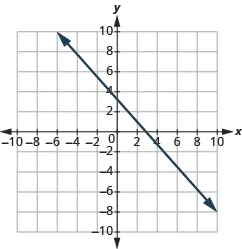118.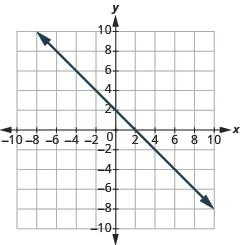119.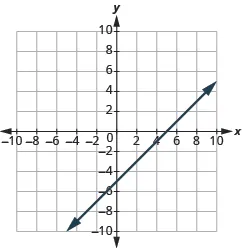120.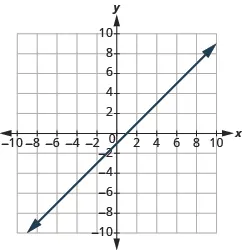121.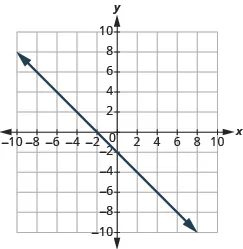122.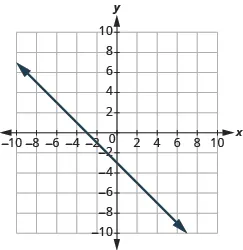123.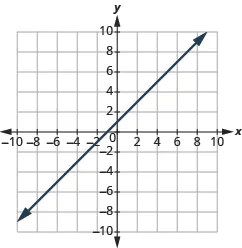124.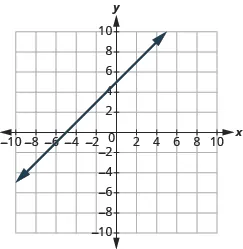125.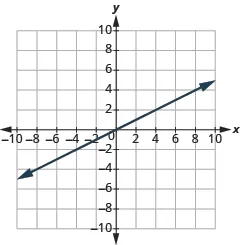126.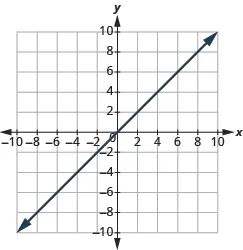Find the $xx$ and $yy$ Intercepts from an Equation of a Line

In the following exercises, find the intercepts.

127.

$x + y = 4 x + y = 4$

128.

$x + y = 3 x + y = 3$

129.

$x + y = −2 x + y = −2$

130.

$x + y = −5 x + y = −5$

131.

$x − y = 5 x − y = 5$

132.

$x − y = 1 x − y = 1$

133.

$x − y = −3 x − y = −3$

134.

$x − y = −4 x − y = −4$

135.

$x + 2 y = 8 x + 2 y = 8$

136.

$x + 2 y = 10 x + 2 y = 10$

137.

$3 x + y = 6 3 x + y = 6$

138.

$3 x + y = 9 3 x + y = 9$

139.

$x −3 y = 12 x −3 y = 12$

140.

$x −2 y = 8 x −2 y = 8$

141.

$4 x − y = 8 4 x − y = 8$

142.

$5 x − y = 5 5 x − y = 5$

143.

$2 x + 5 y = 10 2 x + 5 y = 10$

144.

$2 x + 3 y = 6 2 x + 3 y = 6$

145.

$3 x −2 y = 12 3 x −2 y = 12$

146.

$3 x −5 y = 30 3 x −5 y = 30$

147.

$y = 1 3 x −1 y = 1 3 x −1$

148.

$y = 1 4 x −1 y = 1 4 x −1$

149.

$y = 1 5 x + 2 y = 1 5 x + 2$

150.

$y = 1 3 x + 4 y = 1 3 x + 4$

151.

$y = 3 x y = 3 x$

152.

$y = −2 x y = −2 x$

153.

$y = −4 x y = −4 x$

154.

$y = 5 x y = 5 x$

Graph a Line Using the Intercepts

In the following exercises, graph using the intercepts.

155.

$− x + 5 y = 10 − x + 5 y = 10$

156.

$− x + 4 y = 8 − x + 4 y = 8$

157.

$x + 2 y = 4 x + 2 y = 4$

158.

$x + 2 y = 6 x + 2 y = 6$

159.

$x + y = 2 x + y = 2$

160.

$x + y = 5 x + y = 5$

161.

$x + y = −3 x + y = −3$

162.

$x + y = −1 x + y = −1$

163.

$x − y = 1 x − y = 1$

164.

$x − y = 2 x − y = 2$

165.

$x − y = −4 x − y = −4$

166.

$x − y = −3 x − y = −3$

167.

$4 x + y = 4 4 x + y = 4$

168.

$3 x + y = 3 3 x + y = 3$

169.

$3 x − y = −6 3 x − y = −6$

170.

$2 x − y = −8 2 x − y = −8$

171.

$2 x + 4 y = 12 2 x + 4 y = 12$

172.

$3 x + 2 y = 12 3 x + 2 y = 12$

173.

$3 x −2 y = 6 3 x −2 y = 6$

174.

$5 x −2 y = 10 5 x −2 y = 10$

175.

$2 x −5 y = −20 2 x −5 y = −20$

176.

$3 x −4 y = −12 3 x −4 y = −12$

177.

$y = −2 x y = −2 x$

178.

$y = −4 x y = −4 x$

179.

$y = x y = x$

180.

$y = 3 x y = 3 x$

Choose the Most Convenient Method to Graph a Line

In the following exercises, identify the most convenient method to graph each line.

181.

$x = 2 x = 2$

182.

$y = 4 y = 4$

183.

$y = 5 y = 5$

184.

$x = −3 x = −3$

185.

$y = −3 x + 4 y = −3 x + 4$

186.

$y = −5 x + 2 y = −5 x + 2$

187.

$x − y = 5 x − y = 5$

188.

$x − y = 1 x − y = 1$

189.

$y = 2 3 x −1 y = 2 3 x −1$

190.

$y = 4 5 x −3 y = 4 5 x −3$

191.

$y = −3 y = −3$

192.

$y = −1 y = −1$

193.

$3 x −2 y = −12 3 x −2 y = −12$

194.

$2 x −5 y = −10 2 x −5 y = −10$

195.

$y = − 1 4 x + 3 y = − 1 4 x + 3$

196.

$y = − 1 3 x + 5 y = − 1 3 x + 5$

#### Everyday Math

197.

Road trip Damien is driving from Chicago to Denver, a distance of $1,0001,000$ miles. The $x-axisx-axis$ on the graph below shows the time in hours since Damien left Chicago. The $y-axisy-axis$ represents the distance he has left to drive.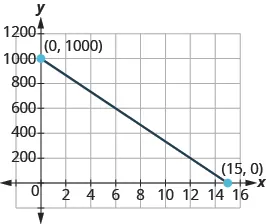Find the $x-x-$ and $y-y-$ intercepts

Explain what the $x-x-$ and $y-y-$ intercepts mean for Damien.

198.

Road trip Ozzie filled up the gas tank of his truck and went on a road trip. The $x-axisx-axis$ on the graph shows the number of miles Ozzie drove since filling up. The $y-axisy-axis$ represents the number of gallons of gas in the truck’s gas tank.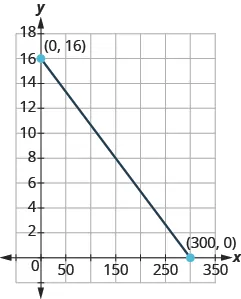Find the $x-x-$ and $y-y-$ intercepts.

Explain what the $x-x-$ and $y-y-$ intercepts mean for Ozzie.

#### Writing Exercises

199.

How do you find the $x-interceptx-intercept$ of the graph of $3x−2y=6?3x−2y=6?$

200.

How do you find the $y-intercepty-intercept$ of the graph of $5x−y=10?5x−y=10?$

201.

Do you prefer to graph the equation $4x+y=−44x+y=−4$ by plotting points or intercepts? Why?

202.

Do you prefer to graph the equation $y=23x−2y=23x−2$ by plotting points or intercepts? Why?

#### Self Check

After completing the exercises, use this checklist to evaluate your mastery of the objectives of this section.

What does this checklist tell you about your mastery of this section? What steps will you take to improve?

Order a print copy

As an Amazon Associate we earn from qualifying purchases.